Next: Hash Joins Up: Sequential Join Algorithms Previous: Brute Force Nested-Loops Joins

## Sort-Merge Joins

As we have seen from the discussion of the nested-loop join, an exhaustive comparison might not be very efficient in many situations. One possibility to avoid this is the following:

1.
Both relations are sorted on the join attributes.
2.
Then, both relations are scanned in the order of the join attributes. Tuples that satisfy the join condition are merged to form the result relation.
This technique is called a sort-merge join.

The concrete sort-merge join algorithm depends on the actual join condition, in the case of a theta-join, for example, on the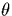operator. Furthermore, it will depend on whether join attributes are keys or not. Let us consider the case of an equi-join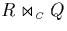with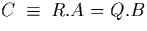with A and B being the integer-valued attributes. The algorithm can then look as shown in figure 3.8. It can be simplified if A or B is a key.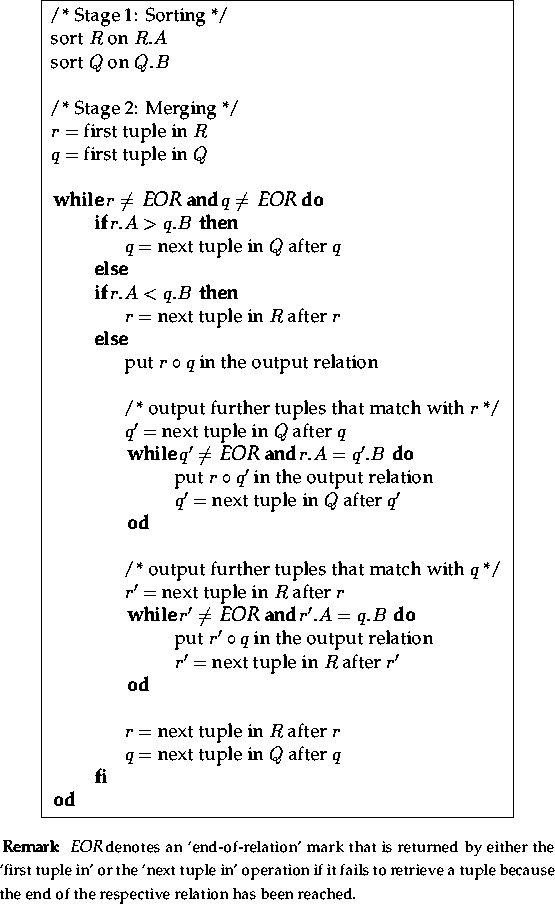The advantage of the sort-merge equi-join is that each relation, if sorted, is scanned only once, thus we have |R| + |Q| tuple accesses on disk compared to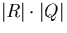for the nested-loop join. Furthermore, the number of unnecessary comparisons is relatively low in any situation. This is very beneficial in the case of a low join selectivity in which many unnecessary comparisons occur when checking all tuples of the cartesian product . This becomes obvious from figure 3.9 which uses the same scenario as figure 3.7 but marks the tuple comparisons performed by the sort-merge equi-join algorithm of figure 3.8. There is only a very small number of mis-hits. The trick behind this strategy is the following: in figure 3.9, the tuples are sorted on the join attribute which implies that the successful comparisons are to be found roughly around the diagonal of the grid (in the case of an equi-join). The sort-merge strategy follows the path along this diagonal and corrects the direction whenever it encounters a mis-hit.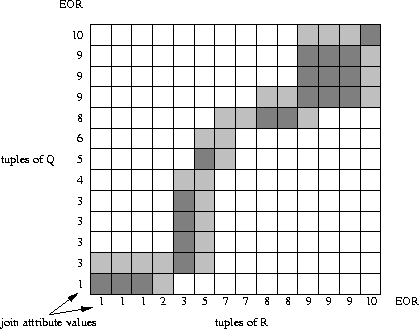The problem of the sort-merge join lies in the requirement that relations have to be sorted on the join attributes prior to the merging stage. In general, this has proved to be the determining component of the execution time [Mishra and Eich, 1992]. If a join needs to be performed frequently for different queries, then the database administrator can choose to sort the table on the join attribute. Many relations, for example, are sorted on their respective key attribute(s). Thus the sorting stage can be omitted for such a relation if the key attribute(s) is also the join attribute(s).

The sort-merge join is fairly robust and is the best choice in many cases, especially when no indices exist over the join attributes [Blasgen and Eswaran, 1977], [Su, 1988].Next: Hash Joins Up: Sequential Join Algorithms Previous: Brute Force Nested-Loops Joins

Thomas Zurek